Chapter 20      Elementary Number Theory

This chapter briefly introduces the basic knowledge of elementary number theory . It is divided into six sections . The first five sections discuss the properties of integers and the method of division , continuous fractions and Fibonacci sequences , congruence and Sun Tzu's theorem , and introduce several important The number-theoretic functions and Mobius transforms of algebraic numbers are listed , and the discrimination methods of several types of irreducible polynomials are listed . The last section gives a brief introduction to the basic concepts and properties of algebraic numbers .

§ 1 Integer

[ Integer part and fractional part ]   Let a be a real number , the largest integer not exceeding a is called the integer part of α , denoted as . And called the fractional part of a .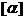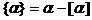For example , , etc.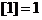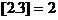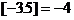The integer part has the following relation :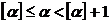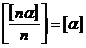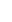, n is a natural number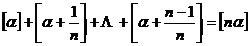, n is a natural number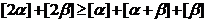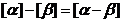or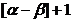Note that the meaning of "rounding operation" in computer programs is different from the meaning of "integer part" here : it was consistent at the time ; it was inconsistent at the time , for example , but after rounding on the computer .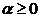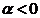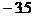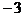[ Divisibility ]   If there is an integer c , such that the integers a and b are suitable for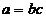Then b is said to be divisible by a , denoted as . In this case, a is called a multiple of b , and b is called a factor ( or submultiple ) of a.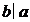If b is not divisible by a , it is recorded as b a .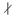Divisibility has the following properties ( the following equations ):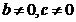1 ° If , , then ;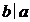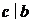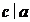2 ° If , then ;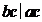3 ° If ​​, , then for any integer m,n we have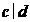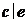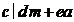4 ° If b is a true factor ( ie ) of a, then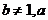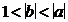[ Prime numbers and Eratos sieve method ] An integer greater than 1 that has exactly 1 and itself two natural numbers as its factors is called a prime number , denoted as . Except for 2 , which is an even prime number , the other prime numbers are odd numbers .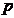Prime numbers have the properties :

There are infinitely many 1 ° prime numbers . If the number of prime numbers not exceeding the natural number n is denoted as p (n) , then at that time , there is * , and further there are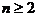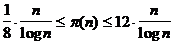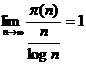2 ° Let p be a prime number , if , then or .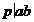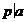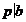The power of the square containing the prime number p in 3 ° is equal to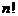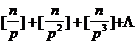4 ° If it is a positive integer , it cannot be divisible by all prime numbers not exceeding , then n must be a prime number . This method of judging whether a natural number is a prime number is called the Eratosian sieve method . This method can establish a prime number table .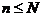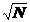[ Unique Decomposition Theorem ] All natural numbers   greater than 1 can be uniquely decomposed into the product of prime powers . If , is a natural number , then n can be uniquely represented as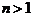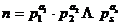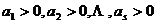( for natural numbers )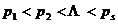( for prime numbers )

This is called the standard decomposition of n . The number s of different prime factors in n does not exceed .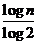Obviously , any natural number n can be expressed as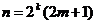( k,m are natural numbers or zero )

This expression is unique .

[ Mason number ]   integer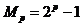( p is a prime number )

Those who are prime numbers are called Meissen numbers . So far only 27 have been found , namely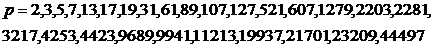Whether there are infinite Meissen numbers has not yet been proved .

[ Fermat number ]   integer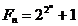( n is a natural number )

It is called the Fermat number . So far, only 5 Fermat numbers have been found to be prime , namely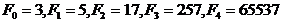None of the following 46 Fermat numbers are prime :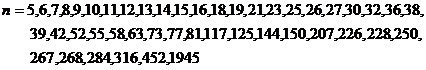[ Reversing and dividing method * ] Each integer a can be uniquely represented by a positive integer b as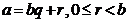In the formula, q is called the incomplete quotient obtained by dividing a by b , and r is called the remainder obtained by dividing a by b . The rolling division method refers to the following finite series of equations :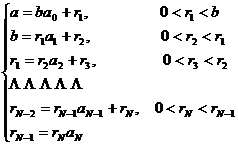( 1 )

Example 1 set a = 525, b = 231, according to the formula (1) , the following formulas and formulas can be listed :

arithmetic grass _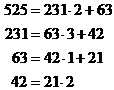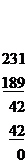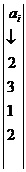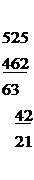[ The greatest common factor and the least common multiple ]   Let a and b be integers . A positive integer that can divide both a and b is called the common factor of a and b , and the largest one is called the greatest common factor of a and b * , denoted as _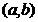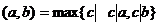Especially at that time , it is said that a and b are mutually prime .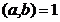Let a and b be positive integers . A positive integer that is divisible by both a and b is called the common multiple of a and b, and the smallest one is called the least common multiple of a and b * , which is written as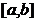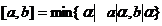Let n positive integers , and define their greatest common factor by induction as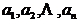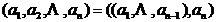Its least common multiple is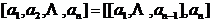The greatest common factor and the least common multiple have the following properties :

1 ° There are integers x, y, such that x , y can be specifically obtained by the rolling division method . It is also obtained by a series of equations of the rolling division method , namely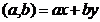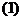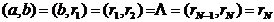Example 2 obtains (525,231)=21 from Example 1. Because the formula from Example 1 has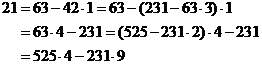So we get x = 4, y = - 9 .

2 ° must exist for any two integers x, y .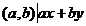3 ° If , , then .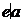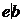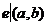4 ° if then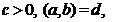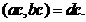If , then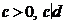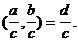5 ° If a and b are two positive integers , they are their prime factors , and the standard decomposition formulas are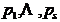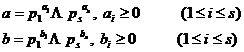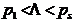but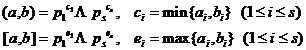6 °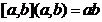If 7 ° is a co-prime positive integer , that is , then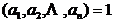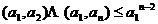* In number theory, the natural logarithm is usuallywritten as.

* Qin Jiushao, an ancient Chinese mathematician(also known as Euclid's algorithm)in "Nine Chapters of the Shushu"1247

* In foreign books and periodicals,the greatest common divisor of a and b is often written as gcd( a , b ),the least common multiple of a and b is often written as lcm ( a , b ).